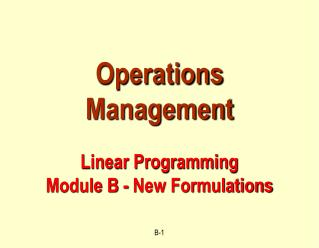DownloadDownload PresentationOperations Management Linear Programming Module B - New Formulations

# Operations Management Linear Programming Module B - New Formulations

Download Presentation## Operations Management Linear Programming Module B - New Formulations

- - - - - - - - - - - - - - - - - - - - - - - - - - - E N D - - - - - - - - - - - - - - - - - - - - - - - - - - -
##### Presentation Transcript

1. New Formulation #1 You are creating an investment portfolio from 4 investment options: stocks, real estate, T-bills (Treasury-bills), and cash. Stocks have an annual rate of return of 12% and a risk measure of 5. Real estate has an annual rate of return of 10% and a risk measure of 8. T-bills have an annual rate of return of 5% and a risk measure of 1. Cash has an annual rate of return of 0% and a risk measure of 0. The average risk of the portfolio can not exceed 5. At least 15% of the portfolio must be in cash. Formulate an LP to maximize the annual rate of return of the portfolio.

2. Constraints:: Average risk  5 At least 15% in cash New Formulation #1 Return Risk Investment 0.12 Stocks 5 0.10 8 Real estate 1 T-bills 0.05 0 Cash 0  5 Portfolio

3. Variables:: xi = % of portfolio in investment type i. i = 1 is Stocks; i = 2 is Real estate; i = 3 is T-bills; i=4 is is Cash New Formulation #1 Return Risk Investment 0.12 Stocks 5 0.10 8 Real estate 1 T-bills 0.05 0 Cash 0  5 Portfolio

4. x4  0.15 (Cash) 5x1 + 8x2 + x3  5 (Risk) x1 + x2 + x3 + x4 = 1.0(Total = 100%) x1, x2, x3 , x40 : Maximize: 0.12x1 + 0.10x2 + 0.05x3 New Formulation #1 xi = % of portfolio in investment type i. i = 1 is Stocks; i = 2 is Real estate; i = 3 is T-bills; i=4 is is Cash Optimal Solution is x1 = 0.85, x2 = 0, x3 = 0 and x4 = 0.15

5. New Formulation #1 xi = % of portfolio in investment type i. Maximize: 0.12x1 + 0.10x2 + 0.05x3 x4  0.15 (Cash) 5x1 + 8x2 + x3  5 (Risk) x1, x2, x3 , x40 Without the total = 100% constraint, the optimal solution is: x1 = 0, x2 = 0, x3 = 5 and x4 = 0.15 This means invest 500% in T-bills and get a 25% return!!

6. Midnight - 4 am 4 am - 8 am 9 3 New Formulation #2 A business operates 24 hours a day and employees work 8 hour shifts. Shifts may begin at midnight, 4 am, 8 am, noon, 4 pm or 8 pm. The number of employees needed in each 4 hour period of the day to serve demand is in the table below. Formulate an LP to minimize the number of employees to satisfy the demand. 8 am - noon Noon - 4 pm 4 pm - 8 pm 8 pm - midnight 15 12 6 13

7. Midnight - 4 am 4 am - 8 am 9 3 Heuristic Solution 8 am - noon Noon - 4 pm 4 pm - 8 pm 8 pm - midnight 15 12 6 13 Start 9 at midnight, then need 0 to start at 4 am, then need 6 to start at 8 am, then need 7 to start at noon, then need 8 to start at 4 pm, and 4 to start at 8 pm. But those 4 also work from midnight to 4 am! Total = 34

8. 12-4 am 4-8 am 8-noon 12-4 pm 4-8 pm 8 pm-12 9 3 6 13 15 12 x1 x2 x3 x4 x5 x6 x6 New Formulation #2 xi = number of employees who start an 8 hour shift at time i i = 1 is midnight, i = 2 is 4 am, i = 3 is 8 am; i=4 is noon, i = 5 is 4 pm, i = 6 is 8 pm

9. New Formulation #2 xi = Number of employees who start an 8 hour shift at time i. i = 1 is midnight, i = 2 is 4 am, i = 3 is 8 am; i=4 is noon, i = 5 is 4 pm, i = 6 is 8 pm Minimize: x1 + x2 + x3 + x4 + x5 + x6 x1 + x6  9 x1 + x2  3 x2 + x3  6 x3 + x4  13 x4 + x5  15 x5 + x6  12 x1, x2, x3 , x4 , x5 , x6 0

10. New Formulation #2 xi = Number of employees who start an 8 hour shift at time i. Minimize: x1 + x2 + x3 + x4 + x5 + x6 x1 + x6  9 Optimal solution = 30 employees x1 = 0, x2 = 3, x3 = 3, x4 = 10, x5 = 5, x6 = 9 x1 = 3, x2 = 0, x3 = 6, x4 = 7, x5 = 8, x6 = 6 x1 + x2  3 x2 + x3  6 x3 + x4  13 x4 + x5  15 x5 + x6  12 x1, x2, x3 , x4 , x5 , x6 0